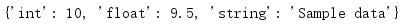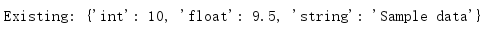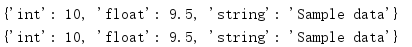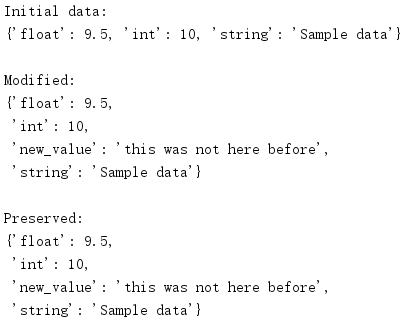# 1. shelve对象的持久存储

## 1.1 创建一个新shelf

import shelve

with shelve.open('test_shelf.db') as s:
s['key1'] = {
'int': 10,
'float': 9.5,
'string': 'Sample data',
}

import shelve

with shelve.open('test_shelf.db') as s:
existing = s['key1']

print(existing)dbm模块不支持多个应用同时写同一个数据库，不过它支持并发的只读客户。如果一个客户没有修改shelf，则可以通过传入flag='r'来告诉shelve以只读方式打开数据库。

import dbm
import shelve

with shelve.open('test_shelf.db', flag='r') as s:
print('Existing:', s['key1'])
try:
s['key1'] = 'new value'
except dbm.error as err:
print('ERROR: {}'.format(err))## 1.2 写回

import shelve

with shelve.open('test_shelf.db') as s:
print(s['key1'])
s['key1']['new_value'] = 'this was not here before'

with shelve.open('test_shelf.db', writeback=True) as s:
print(s['key1'])import shelve
import pprint

with shelve.open('test_shelf.db', writeback=True) as s:
print('Initial data:')
pprint.pprint(s['key1'])

s['key1']['new_value'] = 'this was not here before'
print('\nModified:')
pprint.pprint(s['key1'])

with shelve.open('test_shelf.db', writeback=True) as s:
print('\nPreserved:')
pprint.pprint(s['key1'])## 1.3 特定shelf类型

posted @ 2020-03-23 08:43  SmallGrayCode  阅读(513)  评论(0编辑  收藏  举报